Question

Let X,X,, X, be a random sample of size 3 from a uniform distribution having pdf /(x:0) = θ,0 < x < 0,0 < θ, and let):く,), be the corresponding order statistics. a. Show that 2Y, is an unbi...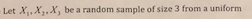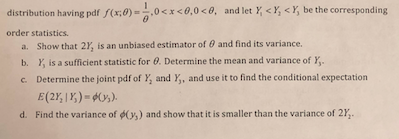Let X,X,, X, be a random sample of size 3 from a uniform
distribution having pdf /(x:0) = θ,0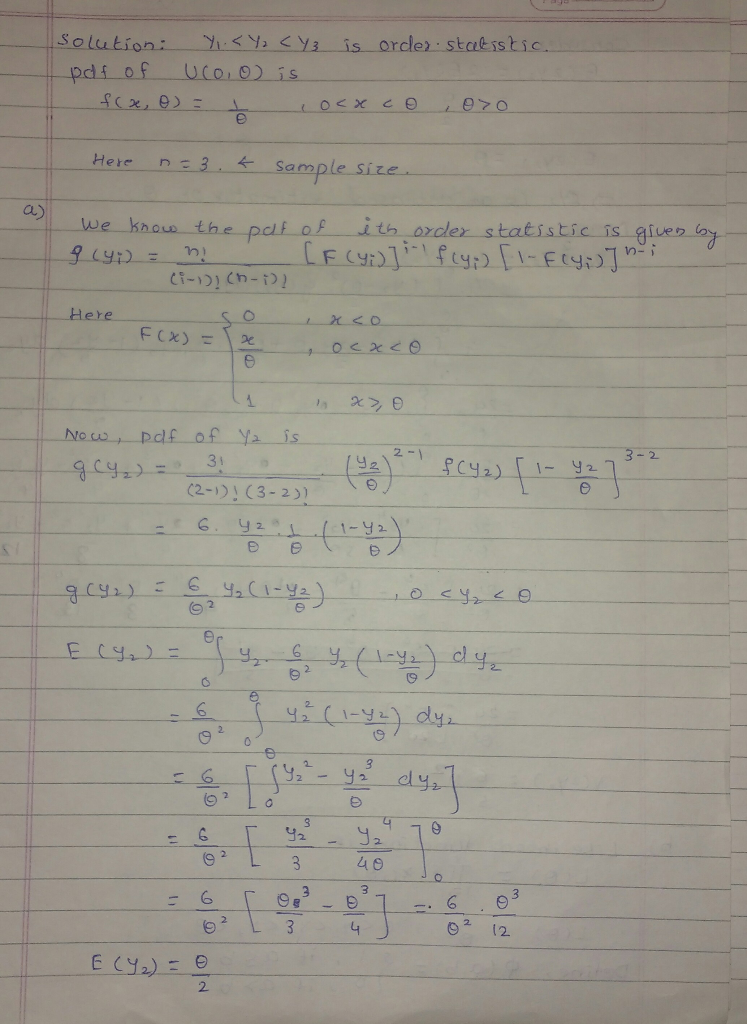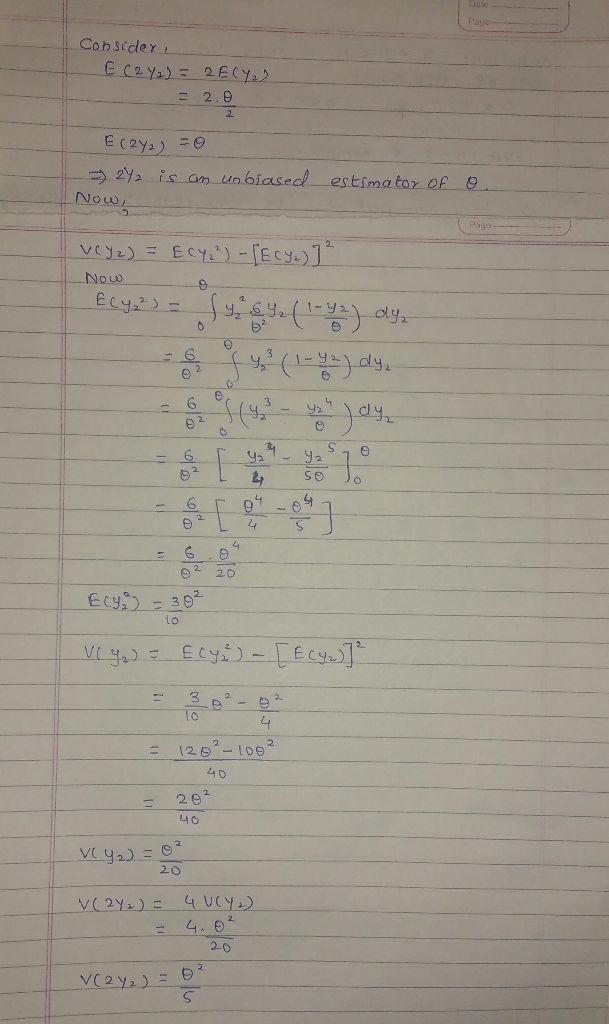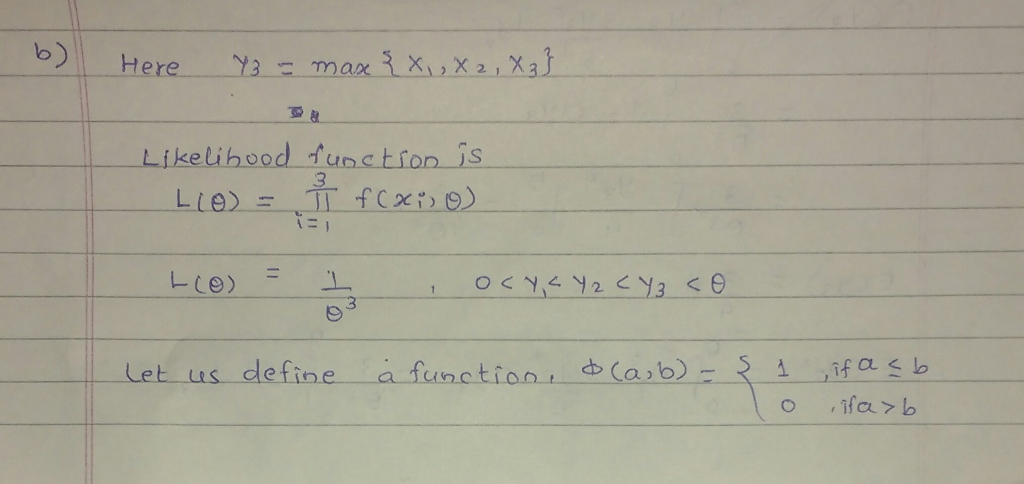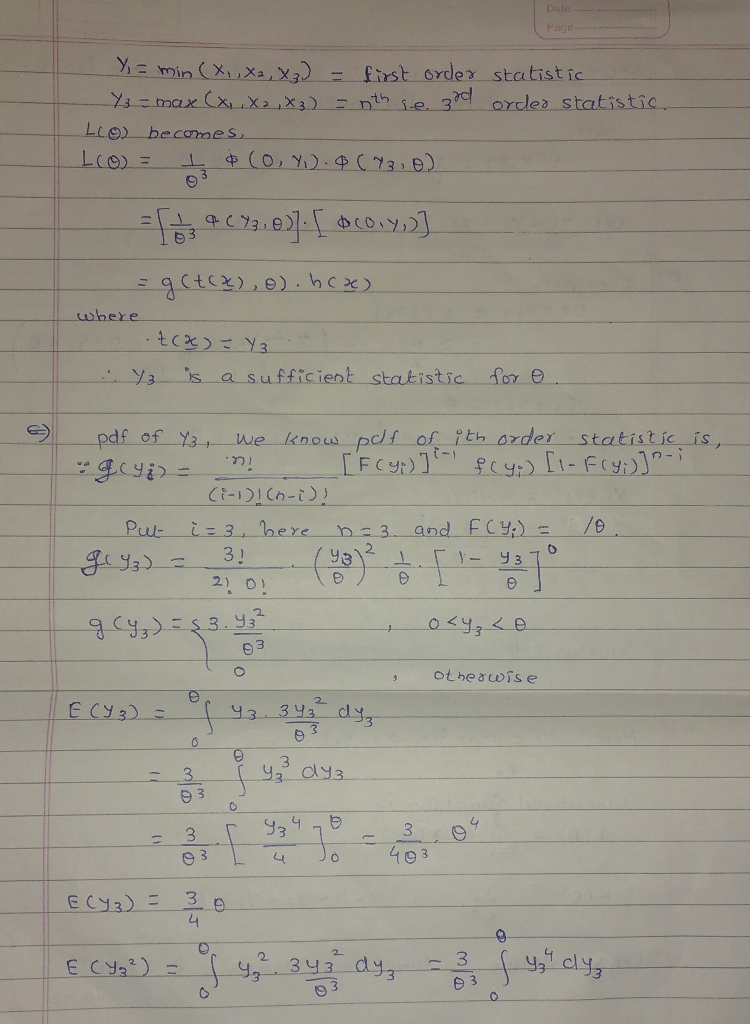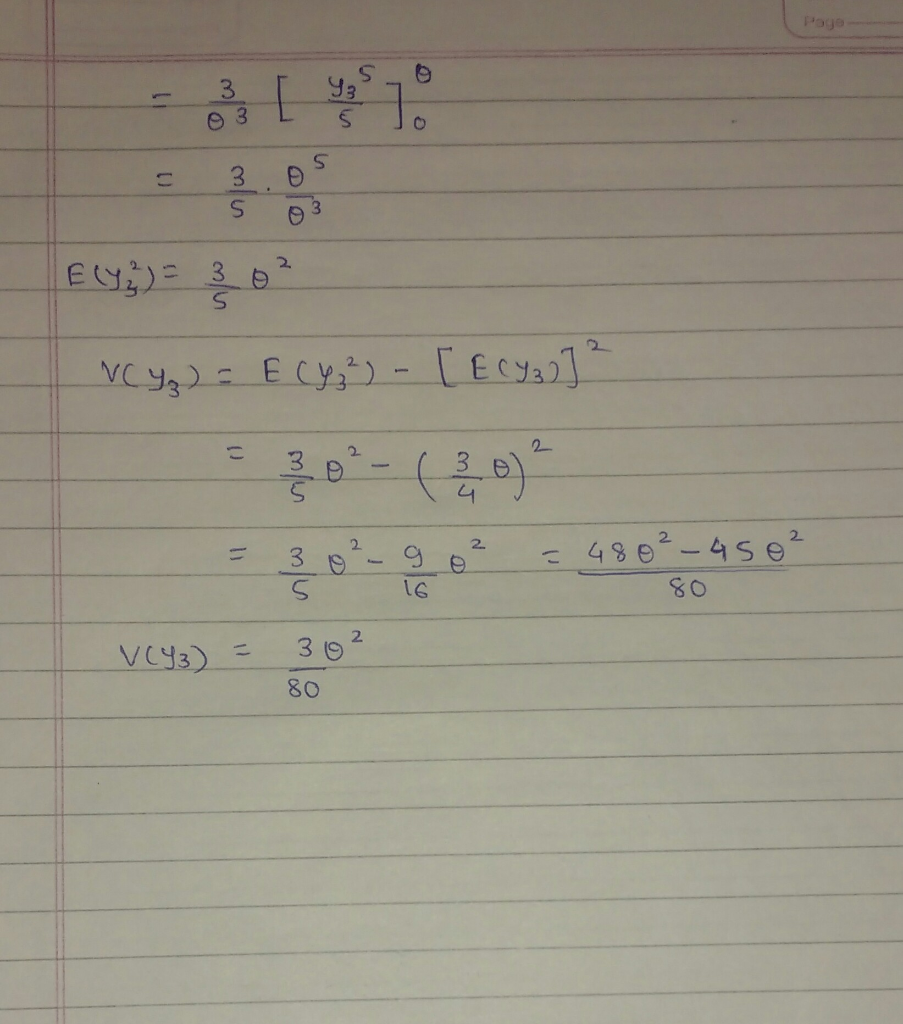Earn Coins

Coins can be redeemed for fabulous gifts.

Similar Homework Help Questions
• Mathematical Statistics แ (Homework y 5) 1. Let , be a random sample fiom the densit where 0 s θ ...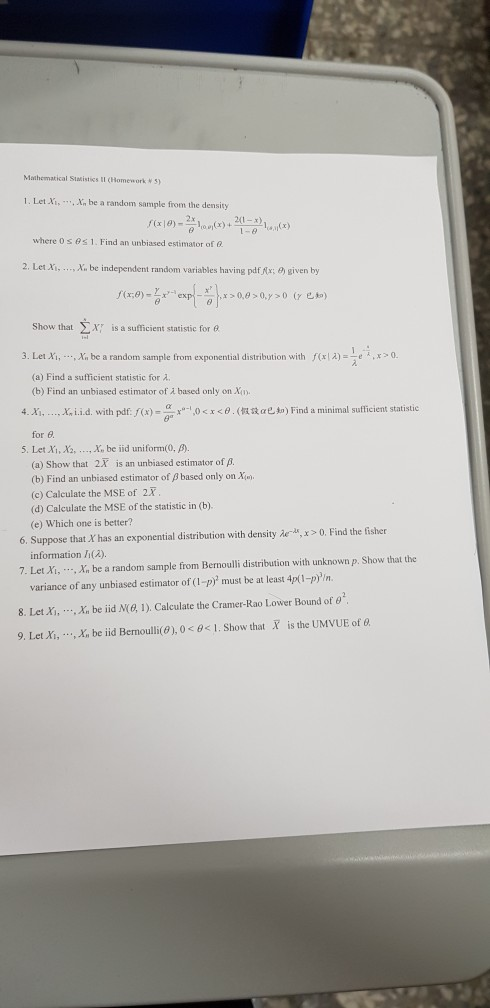difficult…… 2and4   thanks Mathematical Statistics แ (Homework y 5) 1. Let , be a random sample fiom the densit where 0 s θ 1 . Find an unbiased estimator of Q 2. Let Xi, , x. be independent random variables having pdfAx; t) given by Show that X is a sufficient statistic for e f(xl A) =-e- . x > 0 3. Let Xi, , x,' be a random sample from exponential distribution with (a) Find sufficient statistic for λ....

• Mathematical Statistics แ (Homework y 5) 1. Let , be a random sample fiom the densit where 0 s θ ...Mathematical Statistics แ (Homework y 5) 1. Let , be a random sample fiom the densit where 0 s θ 1 . Find an unbiased estimator of Q 2. Let Xi, , x. be independent random variables having pdfAx; t) given by Show that X is a sufficient statistic for e f(xl A) =-e- . x > 0 3. Let Xi, , x,' be a random sample from exponential distribution with (a) Find sufficient statistic for λ. (b) Find an...

• Let X be a random variable with probability density function (pdf) given by fx(r0)o elsewhere where θ 0 is an unknown parameter. (a) Find the cumulative distribution function (cdf) for the random var...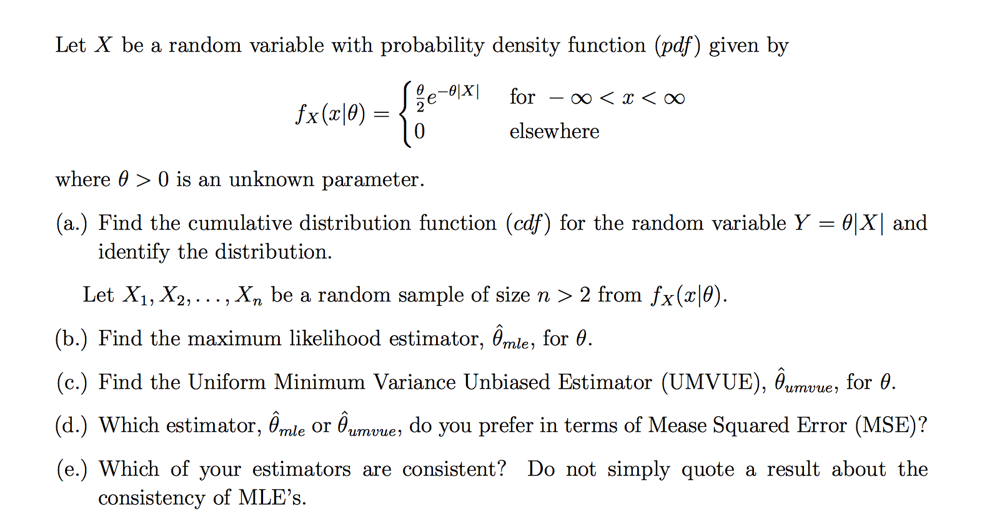Let X be a random variable with probability density function (pdf) given by fx(r0)o elsewhere where θ 0 is an unknown parameter. (a) Find the cumulative distribution function (cdf) for the random variable Y = θ and identify the distribution. Let X1,X2, . . . , Xn be a random sample of size n 〉 2 from fx (x10). (b) Find the maximum likelihood estimator, Ỗmle, for θ (c.) Find the Uniform Minimum Variance Unbiased Estimator (UMVUE), Bumvue, for 0...

• Let Y,,Y.,Y be a random sample of size n from a distribution having pdf a) Show that θ-Ymin is su...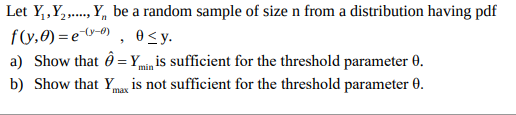Let Y,,Y.,Y be a random sample of size n from a distribution having pdf a) Show that θ-Ymin is sufficient for the threshold parameter θ. b) Show that Ymax is not sufficient for the threshold parameter θ. Let Y,,Y.,Y be a random sample of size n from a distribution having pdf a) Show that θ-Ymin is sufficient for the threshold parameter θ. b) Show that Ymax is not sufficient for the threshold parameter θ.

• 5. Let Yi,Y2, , Yn be a random sample of size n from the pdf (a)...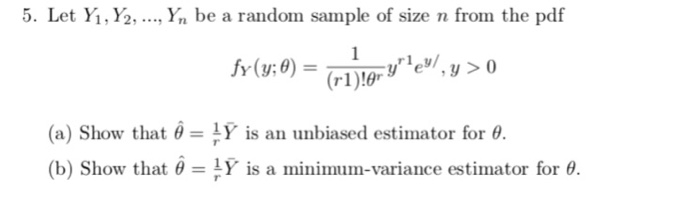5. Let Yi,Y2, , Yn be a random sample of size n from the pdf (a) Show that θ = y is an unbiased estimator for θ (b) Show that θ = 1Y is a minimum-variance estimator for θ.

• 4. Let X,x, X, be a random sample from a uniform distribution on the interval (0,0)...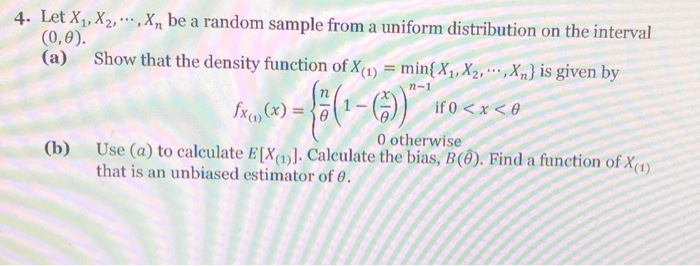4. Let X,x, X, be a random sample from a uniform distribution on the interval (0,0) (a) Show that the density function of XnX,X2 Xn is given by 0 otherwise (b) Use (a) to calculate E[X)). Caleulate the bias, B). Find a function of X) that is an unbiased estimator of .

• , , Yn is a random sample from a distribution with pdf f,0% θ)-22, 3. (20 points) If Y., Y2, 0 Syse, a. find cÝ, where c is a constant, that is an unbiased estimator of θ; and b. show that the varian...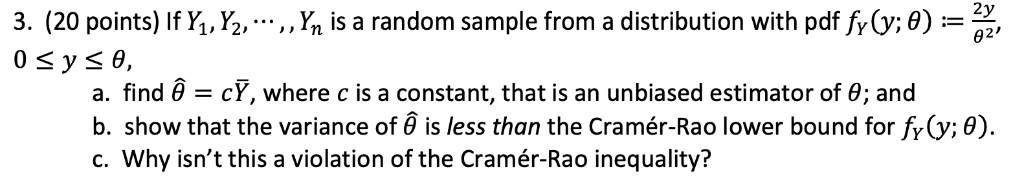, , Yn is a random sample from a distribution with pdf f,0% θ)-22, 3. (20 points) If Y., Y2, 0 Syse, a. find cÝ, where c is a constant, that is an unbiased estimator of θ; and b. show that the variance of is less than the Cramér-Rao lower bound for fr (y; 0) c. Why isn't this a violation of the Cramér-Rao inequality? , , Yn is a random sample from a distribution with pdf f,0% θ)-22, 3....

• Show that the mean X bar of a random sample of size n from a distribution...

Show that the mean X bar of a random sample of size n from a distribution having probability density function f(x;θ)=(1/θ)e-(x/θ) , ,0 < x < ∞ , 0 < θ < ∞ , zero elsewhere, is an unbiased estimator of θ and has variance θ2/n.

• 1. Let Xi,..., Xn be a random sample from a distribution with p.d.f. f(x:0)-829-1 , 0...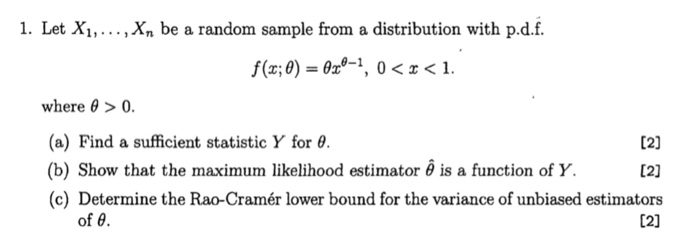1. Let Xi,..., Xn be a random sample from a distribution with p.d.f. f(x:0)-829-1 , 0 < x < 1. where θ > 0. (a) Find a sufficient statistic Y for θ. (b) Show that the maximum likelihood estimator θ is a function of Y. (c) Determine the Rao-Cramér lower bound for the variance of unbiased estimators 12) Of θ

• 10. Let Y1,..., Y, be a random sample from a distribution with pdf 0<y< elsewhere f(x)...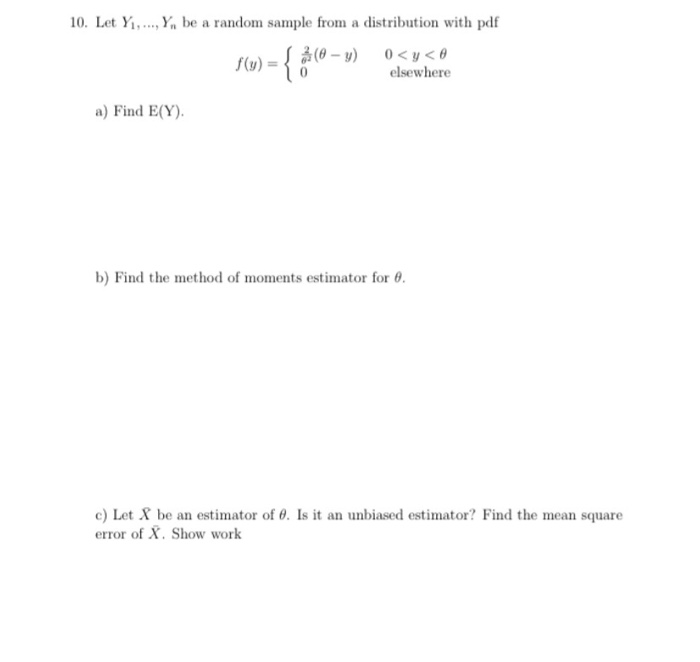10. Let Y1,..., Y, be a random sample from a distribution with pdf 0<y< elsewhere f(x) = { \$(0 –» a) Find E(Y). b) Find the method of moments estimator for 8. c) Let X be an estimator of 8. Is it an unbiased estimator? Find the mean square error of X. Show work